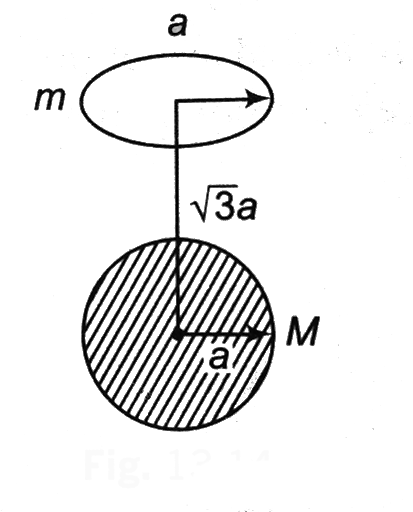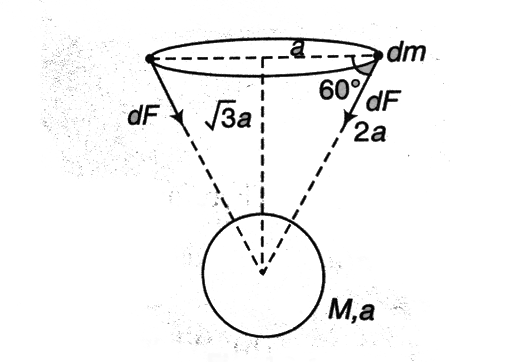A uniform ring of mass m is lying at a distance sqrt(3) a from the | FiloClass 11

Physics

Modern Physics

Gravitation529

A uniform ring of mass m is lying at a distance a from the centre of mass M just over the sphere (where a is the radius of the ring as well as that of the sphere). Find the magnitude of gravitational force between them .Solution: Solution: Net force on ring

as529Connecting you to a tutor in 60 seconds.

Get answers to your doubts.

Similar Topics
simple harmonic motio
modern physics
communication syste
nuclear physic
semiconducting device